# Fractal Wave For Forex Trading (and Stock Market Trading) - page 3

Knowledge is the money in Forex Trading. I hope you have followed well up to the previous post.

In the previous post, we have covered the risk management for the support and resistance.

In fact, what we have covered is for the horizontal support and resistance.

There are other kind of support and resistance, for example, like the diagonal support and resistance.

The great thing is that we can extend the risk management for diagonal support and resistance without adding any complexity.

For your information, the diagonal support and resistance are also those lines that are making up the Triangle Pattern, Rising Wedge Pattern, Falling Wedge Pattern and Channel.

Let us have a look at the simple diagonal support and resistance example.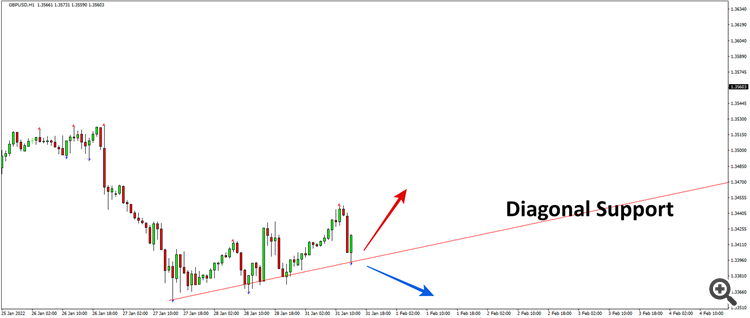If you apply the buy and sell trigger level, the it will look like something like this. As before, as long as you can identify one significant fractal triangle, you can well define your entry, profit target and stop loss.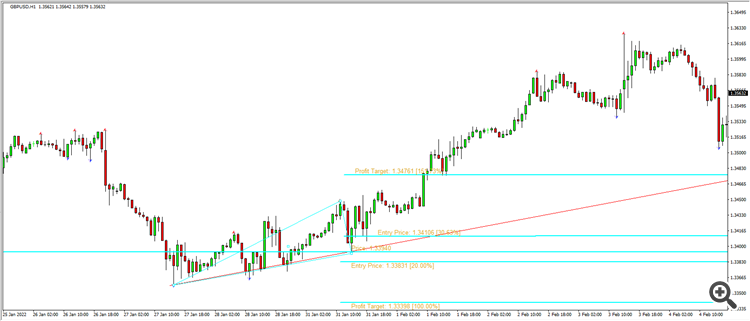However, here are few points I want the newbies to understand. Diagonal support and resistance can be affected by your chart scale.

Depending on your chart scale, the angle of the diagonal support can change.

Hence, I will consider the diagonal support and resistance is slightly harder to trade.

If you are starters, I will recommend mastering the horizontal support and resistance first. After that, go for the diagonal support and resistance or price patterns like  Triangle Pattern, Rising Wedge Pattern, Falling Wedge Pattern and Channel.

Of course, do not forget before using the support and resistance, you need to understand the peak trough analysis.

So far, we made some posts on the market timing and risk management using support and resistance.

The essense is that do not sepearte market timing and the risk managmenet. But do both together in the price chart directly using the support and resistance as well as peak trough analysis.

We tried to make as clear as possible not leaving any gray area when you use the support and resistance for your trading.

Now, one interesting point we can take here is that we can extend what we have learnt for the support and resistance to the supply demand zone trading.

People who just started to use or get used to use the support and resistance may think it is complicated to use the supply and demand zone.

In fact, it is not. We will explain why it is not too complicated.

To start with the check the screenshot. This screenshot will provide the intuitive explantion in the first place.

We have drawn the supply and demand zone in the left and the support and resistance in the right side.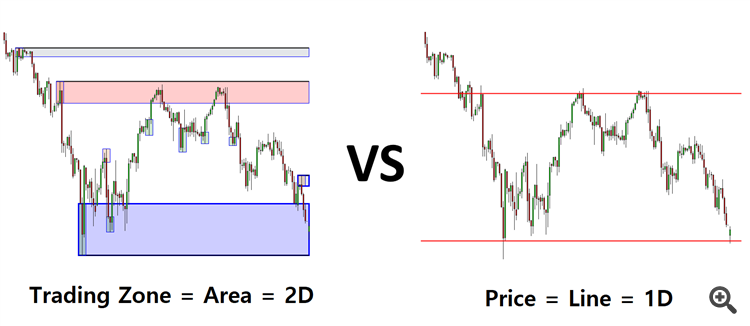When we compare the supply and demand zone to the support and resistance, we are talking about the trading zone against the price level.

In this end, the Supply demand zone can be considered as the support and resistance with the trading zone (=2 dimention = area).

Hence, what we have taught about the market timing for the support and resistance can be used for the supply and demand zone too.

Whatever the indicator is, at first we all have to know that the indicator is formed because of the price, not the other way...

Fractals, Zigzag, even rsi, stochastic or any else, are lagging to the price, YES it can be a good signal or reversal of the price, but you will find many many times in the market that those indicators make a correction to themselves as the price refuse to reverse or continue the movement..

Cheers...

Likewise, consider the harmonic pattern.

In the Harmonic Pattern, the pattern completion interval provides the trading zone like the supply and demand analysis.

Check the screenshot below.

Left and right chart are the same chart.

We have drawn the harmonic pattern in the left chart. We have drawn the support and resistance in the right chart.

In this screenshot, you can feel the trading zone vs price level again for the case of harmonic pattern.

This is an important distinction, between 1 dimension data and 2 dimension data, you can make before you are moving on to the advanced techncial analysis practice.

Many people think the technical anlaysis is complicated.

In fact, no. It is because they have skipped some basics before they go on the the techncial analysis practice they like to use.

As we have shown in this post, one technical anlaysis will provide the basis for the another techncial analysis.

Hence, it is always best to get some organized trading education.

Start from peak through analysis + support and resistance.

Then move onto the more advanced techniques as I have shown in this post.

Opportunity in Forex market is always open as it is a diverse and volatile market

In last post, we covered how trading zone is extended from the line as in support and resistance.

So we will show how the trading zone is used to control your trading risk in place of symmetrical trigger level and symmetrical fibonacci retracment of the support and resistance.

For example, with Pattern Completion Zone (= Pattern Completion Interval), you can set your stop loss and take profit in terms of the height of the Pattern Completion Zone.

In the screenshot below, stop loss is 1 x Pattern Completion Zone and take profit is 3 x Pattern Completion Zone.

In practice, the stop loss can be set little bit wider than 1 x Pattern Completion Zone to avoid any stop hunting.

So you can consider 1 x Pattern Completion Zone as the minimum stop loss size.

Risk management is simple and practical when the Pattern Completion Zone is defined accuartely (Reference: Guide to Precision Harmonic Pattern Trading).

In this post, we tried to demonstrate the idea behind the trading zone. In this case, the trading zone is the pattern completion zone in Harmonic Pattern.

For the risk management example for supply demand zone, it is more or less same as in the case of using the Pattern Completion Zone in Harmonic Pattern Trading (Check the post #26)

For example, this screenshot shows the location of stop loss and target profit for Drop Base Rally Demand Zone.

You are basing the stop loss just below the trough. Of course, you can provide bit more margin below the trough to avoid any stop hunting.

Then you will set your target profit based on the size of your stop loss as shown in the screenshot.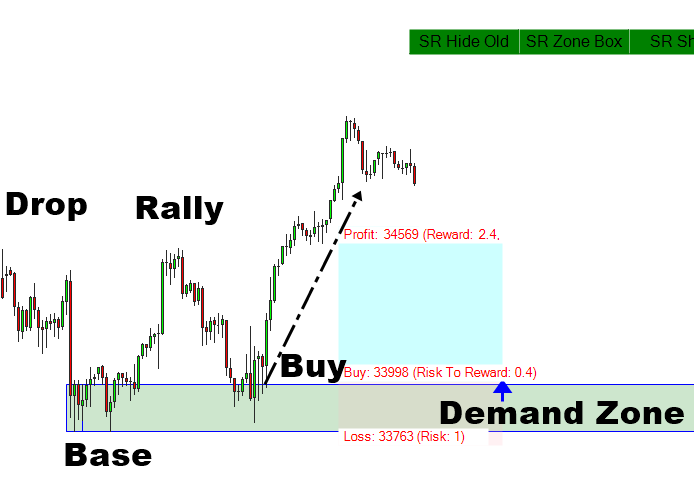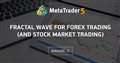• 2022.04.05
• www.mql5.com
Fractal Wave is by far the most conformative and practical theory in the technical analysis. However, it is also most underestimated theory too...

In the past, some people asked me if I use any technical indicators, like the free stuffs generally available in MetaTrader and Tradingview like RSI, Moving Average, etc.

In fact, I will recommend using these techncal indicators as the secondary confirmation only.

In some case, you can even make your trading decision without these techncal indicators.

The direct price analysis or price action study, derived from Fractal wave, should be the primary.

The reason for this is simple.

Say, for example, if you are using these technical indicators as the primary confirmation, then how would set your stop loss and take profit and so on.

To my best knowledge, using 100 pips stop loss and 200 pips take profit or some other fixed value does not make much sense or probably I do not find any rational or logic behind this yet.

This sort of fixed stop and profit target often does not work across different timeframe chart and different symbols.

Even if you trade on one symbol and one timeframe only, there are some period that this fixed stop loss and take profit does not work.

It is hard to predict when it will work or when it will not work.

The simpler but more efficient one is to use peak and trough to set your stop and target and to mark them direclty in your chart.

Market tends to revist the peak or trough multiple times (=market memory).

The respected peak or trough in one timeframe is also respected in another timeframe too.

The direct price analysis or price action study, like the supply demand analysis, harmonic pattern, support resistance, elliott wave analysis, allow you to set your stop and target around peak and trough always.

It is better if you set them around the notable peak and trough.

This is why many experienced trader will recommend the same thing: use direct price analysis or price action study as primary and technical indicators as the secondary confirmation only.

Some people might purely use the direct price analysis or price action study only.

To help your understanding on the market cycle in the financial market, we will break down the complex subject into few simpler one in the context of making buy and sell decision in Forex and Stock market. These 7 points are the essential information you need to understand when you want to make a good buy and sell decision in Forex and Stock Market.

1. Turning Point: a turning point is a price level in which the market can change its direction from bearish to bullish or from bullish to bearish. In fact, the turning point can be considered as the peak and trough in cycle. To visualize it, just consider a simple cycle like a sine curve or cosine curve ranging betweeen 1 and -1 in y axis. Then you can mark the peak at 1 and you can mark the trough at -1 in the curve.
2. Stochastic cycle: Turning point becomes a powerful weapon when you understand the market cycle in Forex and Stock market. However the market cycle is not like a simple cycle like a sine curve or cosine curve. Instead, the market cycle is the stochastic cycle. For example, a sine curve is a determininstic cycle, whose cycle period is fixed. In stochastic cycle, the cycle period is not fixed but probablistic meaning that the cycle period can be alternating around the mean or average.  The harder part is that, there are multiple of stochastic cycles in the market cycle of Forex and Stock market.
3. Fractal Wave: Fractal wave is one tool that can study or predict all the stochastic cycles present in the market in both using statistical and non statistical methods. Fractal wave is simple and effective method proven for many decades along with the evolution of the financial market since Bernoit Mandelbrot dedicated most of his life to the study of fractals, as well as the mathematics of roughness and self-similarity.
4. Fractal Wave for Non statistical method: Fibonacci trading, Support and resistance trading, Harmonic Pattern, Elliott Wave pattern and X3 Chart Pattern can be considered as the chart pattern analysis using the Fractal Wave concept.
5. Fractal Wave for statistical method: Firstly, Fractal Wave theory can be used to perform the Fractal Decomposition technique to extract each market cycle (=stochastic cycle) in Forex and Stock market. Secondly, once the Fractal Decomposition is done, one can visualize each stochastic cycle using Fractal Cycle Analysis.
6. Turning Point Probability: turning point probability is the probability measured in each stochastic cycle extracted and visualized in the Fractal Cycle Analysis to indicate the potential turning point to make a buy or sell decision. Just like other probability, the turning point probability ranges between 0 and 1 or between 0% and 100%.
7. Trend Probability: Although there are many ways to calcualte the trend probability, one can simply calculate the trend probabilty in the Stochastic cycle uisng this simple equation: "the trend probability = 1.0 – the turning point probability".

Since the last post, I have added more complete descriptions towards the term "turning point and trend".  In my opintion + many important literatures in Forex trading, the turning point and trend are the terms you must understand before trading. To give you more insight about turning point and trend in terms of trading strategy, we have summarized the important terms as follows:

1. Trends: a trend is a tendency for prices to move in a particular direction over a period. Depending on its period, trends can be short term, med term and long term. Depending on its direction, trends can be categorized into upward trends, downward trends and even sideways.
2. Turning Point (General Term): A turning point is a price point from which a minor or major trend reversal happens.
3. Turning Point (Scientific Term): A turning point is a price level in which the market can change its direction from bearish to bullish or from bullish to bearish. In fact, the turning point can be considered as the peak and trough in cycle. To visualize it, just consider a simple cycle like a sine curve or cosine curve ranging betweeen 1 and -1 in y axis. Then you can mark the peak at 1 and you can mark the trough at -1 in the curve.
4. Stochastic cycle: Turning point becomes a powerful weapon when you understand the market cycle in Forex and Stock market. However the market cycle is not like a simple cycle like a sine curve or cosine curve. Instead, the market cycle is the stochastic cycle. For example, a sine curve is a determininstic cycle, whose cycle period is fixed. In stochastic cycle, the cycle period is not fixed but probablistic meaning that the cycle period can be alternating around the mean or average.  The harder part is that, there are multiple of stochastic cycles in the market cycle of Forex and Stock market.
5. Fractal Wave: Fractal wave is one tool that can study or predict all the stochastic cycles present in the market in both using statistical and non statistical methods. Fractal wave is simple and effective method proven for many decades along with the evolution of the financial market since Bernoit Mandelbrot dedicated most of his life to the study of fractals, as well as the mathematics of roughness and self-similarity.
6. Fractal Wave for Non statistical method: Fibonacci trading, Support and resistance trading, Harmonic Pattern, Elliott Wave pattern and X3 Chart Pattern can be considered as the chart pattern analysis using the Fractal Wave concept.
7. Fractal Wave for statistical method: Firstly, Fractal Wave theory can be used to perform the Fractal Decomposition technique to extract each market cycle (=stochastic cycle) in Forex and Stock market. Secondly, once the Fractal Decomposition is done, one can visualize each stochastic cycle using Fractal Cycle Analysis.
8. Turning Point Probability: turning point probability is the probability measured in each stochastic cycle extracted and visualized in the Fractal Cycle Analysis to indicate the potential turning point to make a buy or sell decision. Just like other probability, the turning point probability ranges between 0 and 1 or between 0% and 100%.
9. Trend Probability: Although there are many ways to calcualte the trend probability, one can simply calculate the trend probabilty in the Stochastic cycle uisng this simple equation: “the trend probability = 1.0 – the turning point probability”.

As you can see, two more points are added. Hope this helps everyone.

Reason: